Definition: construction from The Penguin Dictionary of Mathematics

The process of drawing a given geometric figure; for example, the construction of a line at right angles to a given line or the construction of a line bisecting a given angle. Usually, it is required that this be done using only compasses and straightedge. The three classical constructions of antiquity, dating from the 4th century BC, are squaring the circle, duplication of the cube, and trisection of an angle. Mascheroni constructions are ones that require only compasses. See also Fermat numbers.

Summary Article: construction
From The Hutchinson Unabridged Encyclopedia with Atlas and Weather Guide

In geometry, an addition to a figure, which is drawn to help solve a problem or produce a proof. The term is also applied to the accurate drawing of shapes.

Construction of basic shapes and angles can be carried out using a ruler, protractor, and compasses.

Construction of a perpendicular bisector to a line The compasses are opened to a little more than half the length of the line – this distance is kept throughout the construction. The compass point is placed first on one end of the line and then on the other, and arcs drawn to meet above and below the line. A perpendicular line can be drawn through the meeting points: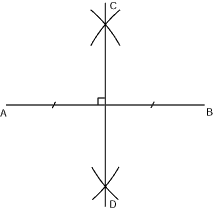The line CD bisects the line AB at right angles.

Construction of parallel lines a specified distance apart The required distance is measured (A) and point P marked anywhere on the line. The compasses are opened to the length AP – this distance is kept throughout the construction. With the compass point placed on P, an arc is drawn to cut the line (point Q). Then with the compass point first on Q and then on A, two arcs are drawn to cross at a point. The line drawn through A and the meeting point is parallel to the original line: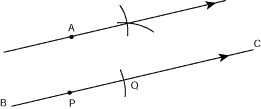Construction of the bisector of any angle The compasses are opened to a length less than that of either arm of the angle – this distance is kept throughout the construction. With the compass point on the point of the angle, arcs are drawn to cut each arm (points A and B). Then with the compass point first on A and then on B, two arcs are drawn to cross at a point. The line drawn from this meeting point to the point of the angle cuts the original angle into two equal angles: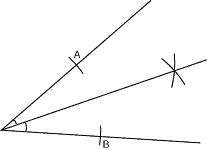Constructing trianglesTriangles may be constructed from a variety of given measurements using a ruler, compass, and protractor.

Given three sides (SSS): triangle ABC can be constructed knowing that AB = 8 cm, BC = 7 cm, and AC = 6 cm.

First AB is drawn, 8 cm long. Then with the compasses centred on A, radius 6 cm, an arc is drawn. The compasses are extended to 7 cm and, centring on B, another arc is drawn to cross through the first arc at C. AC and BC are then joined to create the triangle ABC:Given one side and two angles (ASA): triangle ABC can be constructed knowing that AB = 6 cm, angle A = 36°, and angle B = 59°.

The line AB is drawn, 6 cm long. A protractor is used to measure the angles 36° at A and 59° at B – these lines are continued to meet at C, forming the triangle ABC: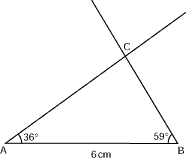Given two sides and the angle between them (SAS): triangle ABC can be drawn knowing that AB = 5 cm, angle A = 45°, and AC = 4 cm.

Line AB is drawn, 5 cm long. An angle of 36° is measured at A, and the arm extended to a length of 4 cm to give point C. Points B and C are joined to form the triangle: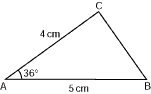Given two sides and a non-included angle (SSA): triangle ABC can be drawn knowing that AB = 6 cm, BC = 7 cm, and angle A = 50°.

Line AB is drawn, 6 cm long. An angle of 50° is measured at A and the arm extended. With the compasses centred on B, radius 7 cm, an arc is drawn to cross the extended line at C. Points B and C are joined to form the triangle:© RM, 2018. All rights reserved.

### Related Articles

##### Full text Article Construction
Miller's Antiques Encyclopedia

Early furniture was primitively constructed out of the solid, often re-using old pieces of timber. During the 17th and 18th centuries,...

See more from Credo